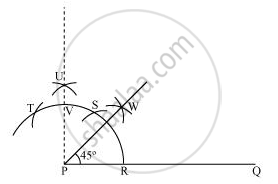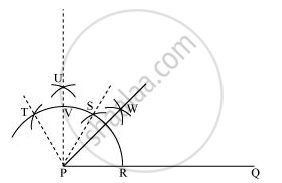Share

# Construct an angle of 45° at the initial point of a given ray and justify the construction. - CBSE Class 9 - Mathematics

ConceptBasic Constructions

#### Question

Construct an angle of 45° at the initial point of a given ray and justify the construction.

#### Solution

The below given steps will be followed to construct an angle of 45°.

(i) Take the given ray PQ. Draw an arc of some radius taking point P as its centre, which intersects PQ at R.

(ii) Taking R as centre and with the same radius as before, draw an arc intersecting the previously drawn arc at S.

(iii) Taking S as centre and with the same radius as before, draw an arc intersecting the arc at T (see figure).

(iv) Taking S and T as centre, draw an arc of same radius to intersect each other at U.

(v) Join PU. Let it intersect the arc at point V.

(vi) From R and V, draw arcs with radius more than 1/2RV to intersect each other at W. Join PW.

PW is the required ray making 45° with PQ.Justification of Construction:

We can justify the construction, if we can prove ∠WPQ = 45°.

For this, join PS and PT.We have, ∠SPQ = ∠TPS = 60°. In (iii) and (iv) steps of this construction, PU was drawn as the bisector of ∠TPS.

∴ ∠UPS = 1/2 ∠TPS = 60°/2 = 30°

Also, ∠UPQ = ∠SPQ + ∠UPS

= 60° + 30°

= 90°

In step (vi) of this construction, PW was constructed as the bisector of ∠UPQ.

∴ ∠WPQ = 1/2 ∠UPQ = 90°/2 = 45°

Is there an error in this question or solution?

#### APPEARS IN

NCERT Solution for Mathematics Class 9 (2018 to Current)
Chapter 11: Constructions
Ex. 11.10 | Q: 2 | Page no. 191

#### Video TutorialsVIEW ALL 

Solution Construct an angle of 45° at the initial point of a given ray and justify the construction. Concept: Basic Constructions.
S